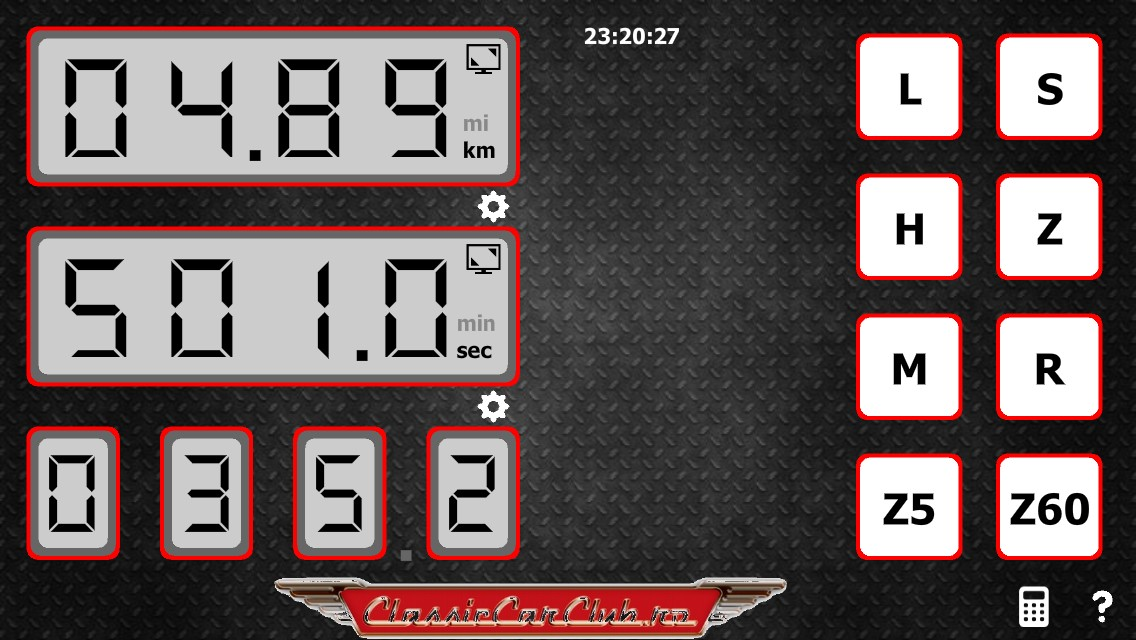Speed Table Calculator

Rally Speed Table Calculator is the perfect helper for your next regularity, tsd, historic or classic rally.

It's designed to help the pilots to respect the average speeds during regularity rallying, also known as TSD rallying (Time, Speed and Distance). An additional feature of the app is to calculate seconds based on given speed and distance.

Rally Speed Table Calculator allows you to control your advance or delays:
 the application displays in real time the theoretical distance to run to keep the imposed average speed while it's shown the chronometer also.

Basic Functionalities:
- calculate the theoretical distance based on speed and time elapsed
- countdown for starting the section from 5 and 60 seconds
- light function
- beeps on 100m or 1km
- works with in miles as well as in kilometers
- recalculate the distance based on modified speed
- modify the speed during a section
- full screen mode

Calculator functionalities:
- calculate the speed based on distance and time
- calculate the distance based on speed and time
- calculate the time based on speed and distance

Dont hesitate, give it a try with the free version, limited some functionalities.FREE14.99Euro
- set the speed of the section with 100m punctuality
- sound functionality
- light functionality
- full screen
- seconds and minutes
- modify the time of the section
- R modify the speed during the section
- M recalculate the distance
- countdown from 5 and 60 seconds
- calculator functionality
- help page